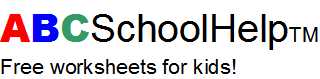Back | Print page | Home

# Grade 1 Math: Addition and Subtraction 6

```a.  Use 5, 3, and 2 to make two correct number sententces using addition

____ + ____ = ____

____ + ____ = ____

b.  Use 8, 2, and 6 to make two correct number sententces using addition

____ + ____ = ____

____ + ____ = ____

c.  Use 8, 2, and 6 to make two correct number sententces using subtraction

____ - ____ = ____

____ - ____ = ____
```